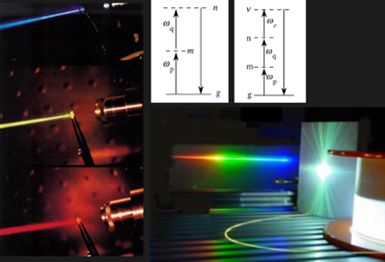# OSE6334 - Nonlinear OpticsMaxwell’s equations in linear and nonlinear media, 2nd and 3rd -order optical nonlinearities, nonlinear crystals, phase-matching, frequency conversion techniques, stimulated scattering, self-phase modulation, pulse compression, solitons.

This course studies the interaction of intense light with optically transparent media when the interaction becomes non-linear, that is, when Newton’s principle of superposition ceases to operate. Starting with Maxwell’s equations, the course develops the formalism of nonlinear optics, introducing the concept of nonlinear susceptibility, explained using both a simple mechanical analogy and quantum mechanical perturbation theory. The goal is to equip students with knowledge about non-linear effects of different (2nd, 3rd, etc.) orders, about non-linear materials, and how they can apply non-linear optical effects to various experimental scenarios.

Pre-requisites:  Graduate Standing and Consent of Instructor

• R. W. Boyd, Nonlinear Optics, 4-th Ed. (Acad. Press, 2020)
• G.I. Stegeman, R.A. Stegeman, Nonlinear Optics Phenomena, Materials, and Devices (Wiley, 2012)

Topics

• Maxwell’s equations in nonlinear media
• Nonlinear susceptibility based on a classical anharmonic oscillator analogy
• Nonlinear susceptibility based on the quantum-mechanical perturbation theory
• Coupled-wave equations for nonlinear optical interactions
• Nonlinear optical crystals with 2nd-order susceptibility
• Phase matching in nonlinear optical interactions
• Quasi-phase-matching (QPM)
• Sum-frequency and 2nd-harmonic generation. Difference-frequency generation.
• Conversion efficiency of nonlinear optical processes
• Optical parametric oscillators (OPOs) and amplifiers (OPAs)Tuning curves.
• Nonlinear effects inside a resonant cavity
• Nonlinear optics with ultrafast optical pulses.
• 3rd-order nonlinear susceptibility ?(3) and its tensor representation
• Kerr effect. Intensity-dependent refractive index. Self-focusing. Self-phase modulation.
• Parametric processes due to 4-wave mixing. 3rd harmonic generation.
• Phase conjugation, two-photon absorption, all-optical switching
• Techniques for measuring 2nd and 3rd -order nonlinearities. Z-scan.
• Stimulated Brillouin scattering. Stimulated Raman scattering